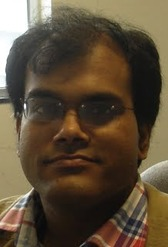Dr. K. GongopadhyayProfessor, Mathematical Sciences Email krishnendu(AT)iisermohali.ac.inPhone +91 172 2293101 Fax +91 172 2240266 Personal Page ORCID ID 0000-0003-4327-0660 Research AreaGroups and Geometry
 Research Focus I have worked on the following research themes: (i) Algebraic classification of the isometries in hyperbolic geometries, and (ii) to classify the z-classes in classical groups.Classically, the isometries of the two dimensional real hyperbolic space are classified algebraically in terms of their traces. An interesting problem is to generalize this classification in the higher dimensions, as well as in the geometry of other rank one symmetric spaces of non-compact type. I have obtained satisfactory answer to this problem especially in (i) real hyperbolic geometry up to dimension 5 and, (ii) low dimensional complex and quaternionic hyperbolic geometries.Let G be a group. For x, y in G we say x and y belong to same z-class if their centralizers ZG(x) and ZG(y) are conjugate in G. The z-classes in a group give a partition of G, and they refine the partition of G into the conjugacy classes. R. S. Kulkarni has proposed that this notion may be used to make precise the intuitive idea of “dynamical types" in any “geometry", whose automorphism group contains a copy of G. When the z-classes provide a finite partition of the automorphism group, algebraically it gives another characterization of the isometries. In this sense the above themes (i) and (ii) are related from the geometric point of view. Apart from geometric interest, an interesting problem in its own right is to classify the conjugacy and the z-classes in a given group. We have classified the z-classes in hyperbolic geometries. A better understanding of the conjugacy classes in real hyperbolic geometry has also been obtained. Efforts are underway to understand the z-classes in orthogonal and symplectic groups over arbitrary fields. Some closely related problems are also worked out. Selected Publications K. Gongopadhyay and W. S. Cao, Proc. Amer. Math. Soc. (to appear.) Commuting isometries of the complex hyperbolic space. K. Gongopadhyay, Geom. Dedicata, DOI: 10.1007/s10711-010-9531-6. Conjugacy classes in M\"obius groups. K. Gongopadhyay and Ravi S. Kulkarni, inear Algebra and its Applications. Volume 434, Issue 1 (2011), 89--103. On the existance of invariant non-degenerate bilinear form under a linear map. K. Gongopadhyay, Expo. Math. Volume 28, Issue 4 (2010), 351-356. On the conjugacy classes in the orthogonal and symplectic groups over algebraically closed fields. K. Gongopadhyay, Geom. Dedicata, Volume 144, Issue 1 (2010), Page 157--170. Algebraic characterization of the isometries of the hyperbolic 5-space. K. Gongopadhyay and Ravi S. Kulkarni, Conform. Geom. Dyn. 13 (2009), 91—109. z-Classes of isometries of the hyperbolic space.